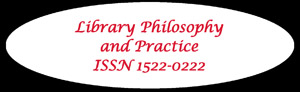## Libraries at University of Nebraska-Lincoln## Library Philosophy and Practice (e-journal)

Summer 7-12-2019

### Abstract

Purpose - The purpose of this study is to inspect the mobile reading behaviors and readers’ content predilection in Hyderabad city, India and to find out the interrelated problems.

Design/methodology/approach - This study used a survey method and questionnaire tool for collecting data. Simple random sampling technique is employed to determine the sample population. SPSS software is used for analyzing data, independent t-test and One-Way ANOVA tests are conducted to test the stated hypotheses.

Findings - The findings from t-test results discloses that there is a significant difference concerning males and females in term of reading e-books (t-value = -2.570, p-value = 0.011), particularly stories/novels (t-value = -4.021, p-value = 0.001), and time spent for emails (t-value = -3.394, p-value = 0.001). Also there is a significant difference concerning occupation and in terms of reading online magazines (t-value = -2.181, p-value = 0.033), e-books (t-value = -2.357, p-value = 0.019), stories/novels (t-value = -3.423, p-value = 0.001), and time spent on emails (t-value = -2.151, p-value = 0.032). The ANOVA test results shows that online news has significant difference (F-value = 2.727, p-value = 0.030) in term of age groups and used of e-books (F-value = 4.149, p-value = 0.007), using emails (F-value = 2.807, p-value = .040), movie reviews (F-value = 5.738, p-value = 0.001) and comic strips or jokes (F-value = 5.896, p-value = 0.001) have significant difference in term of their educational qualification(s).

Originality/value - This paper help to comprehend the choice of reading a different type of contents based on demographic dissimilarities.